Courses

# RD Sharma Solutions - Ex-5.2, Operations On Rational Numbers, Class 7, Math Class 7 Notes | EduRev

## Class 7 : RD Sharma Solutions - Ex-5.2, Operations On Rational Numbers, Class 7, Math Class 7 Notes | EduRev

The document RD Sharma Solutions - Ex-5.2, Operations On Rational Numbers, Class 7, Math Class 7 Notes | EduRev is a part of the Class 7 Course RD Sharma Solutions for Class 7 Mathematics.
All you need of Class 7 at this link: Class 7

Q1. Subtract the first rational number from the second in each of the following:

Solution.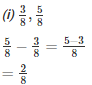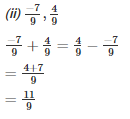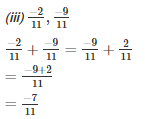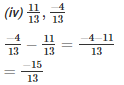Q2. Evaluate each of the following:

Solution.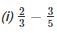LCM of 3 and 5 is 15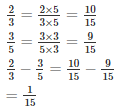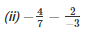LCM of 3 and 7 is 21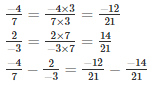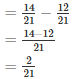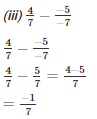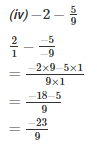Q3. The sum of the two numbers is 5/9. If one of the numbers is 1/3, find the other.

Solution.

Required number =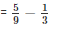LCM of 3 and 9 is 9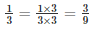Therefore required number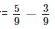=  2/9

Q4. The sum of two numbers is −1/3. If one of the numbers is −12/3, find the other.

Solution.

Let the required number be x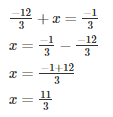The required number is 11/3

Q5. The sum of two numbers is −4/3. If one of the numbers is -5, find the other.

Solution.

Let the required number be x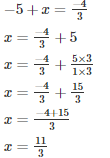The required number is 11/3

Q6. The sum of two rational numbers is -8. If one of the numbers is −15/7, find the other.

Solution.

Let the required number be x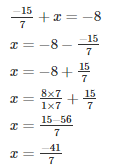The required number is -41/7

Q7. What should be added to −7/8 so as to get 5/9?

Solution.

Let the required number be x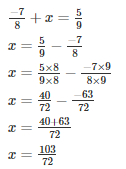The required number is 103/72

Q8. What number should be added to -5/11 so as to get 26/33?

Solution.

Let the required number be x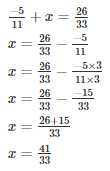The required number is 41/33

Q9. What number should be added to −5/7 to get −2/3?

Solution.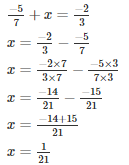The required number is 1/21

Q10. What number should be subtracted from −5/3 to get 5/6?

Solution.

Let the required number be x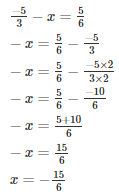The required number is 15/6

Q11. What number should be subtracted from 3/7 to get 5/4?

Solution.

Let the required number be x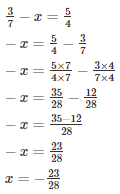The required number is 23/28

Q12. What should be added to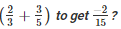Solution.

Let the required number be x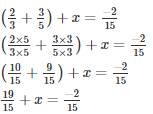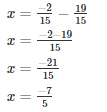The required number is −7/5

Q13. What should be added toSolution.

Let the required number be xThe required number is 59/30

Q14. What should be subtracted from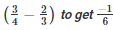Solution.

Let the required number be x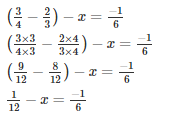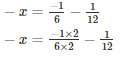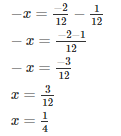The required number is 1/4

Q15. Simplify:

Solution.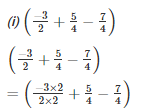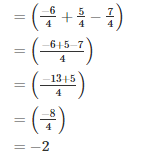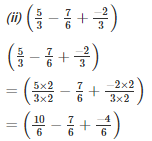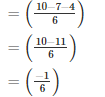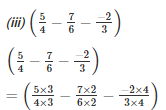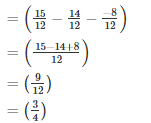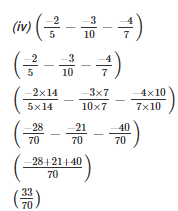Q16. Fill in the blanks:

Solution.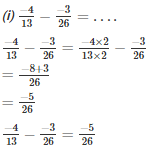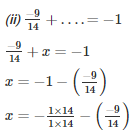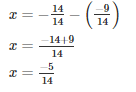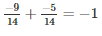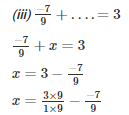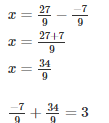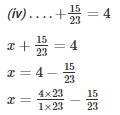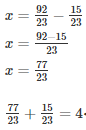Offer running on EduRev: Apply code STAYHOME200 to get INR 200 off on our premium plan EduRev Infinity!

97 docs

,

,

,

,

,

,

,

,

,

,

,

,

,

,

,

,

,

,

,

,

,

,

,

,

,

,

,

,

,

,

;